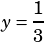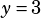4x + y = 7
2x – 7y = 1

If I multiply the second equation by 2, I can stack them and subtract:

4x + y = 7
4x – 14y = 2

So, 15y = 5, —> y = 3

Then: 2x – 7(3) = 1 –> 2x – 21 = 3 —> 2x = 24 –> x = 12
But: 4x + 3 = 7 –> 4x = 4 –> x = 1

What am I not seeing? The answer should be x= 5/3.

Careful!simplifies to, not.Robin says: